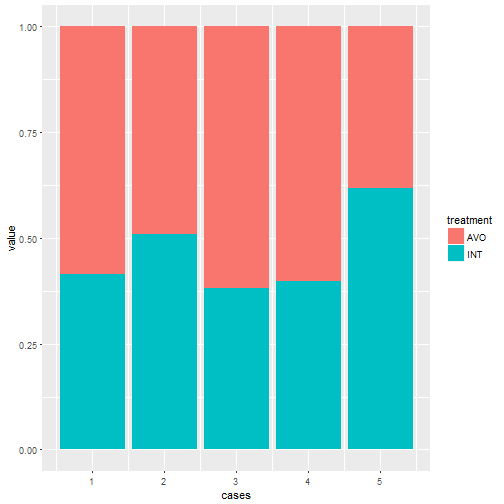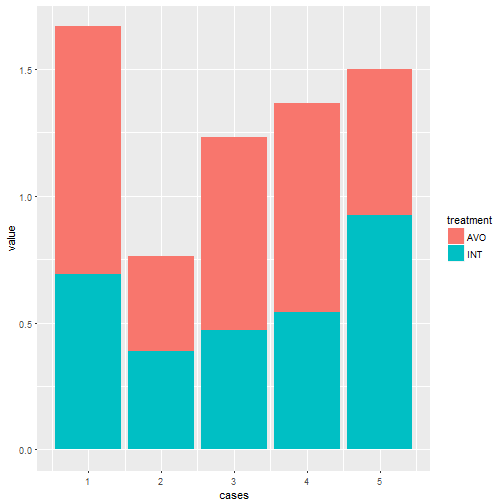# Barcharts to percentages

Maybe not the most correct headline.

Anyway. Assume we have five cases, with two values for a given treatment, each labelled “AVO” and “INT”. How to get a barchart, where each cases is one bar, and the bars are coloured based on the percentages of the two values?

Lets make some sample data:

``````set.seed(47)
cases <- 1:5
cases <- c(cases, cases)
treat1 <- rep("AVO", 5)
treat2 <- rep("INT", 5)
treat <- c(treat1, treat2)
val <- runif(10)

df <- data.frame(cases=cases, treatment = treat, value = val, stringsAsFactors = FALSE)
``````

How does that look?

``````df
``````
```##    cases treatment     value
## 1      1       AVO 0.9769620
## 2      2       AVO 0.3739160
## 3      3       AVO 0.7615020
## 4      4       AVO 0.8224916
## 5      5       AVO 0.5735444
## 6      1       INT 0.6914124
## 7      2       INT 0.3890619
## 8      3       INT 0.4689460
## 9      4       INT 0.5433097
## 10     5       INT 0.9248920
```

OK, nice and tidy data.

What I want is a bar for case 1, filled with percentages. One color with 0.976/(0.976+0.691) and another color for 0.691/(0.976+0.691).

``````library(ggplot2)
ggplot(df)+
geom_col(aes(y=value, x=cases, fill=treatment), position = "fill")
``````We’re using geom_col. That is a shorthand for geom_bar with stat=“identity”, and is the shortcut to expressing values in the barchart.

The y-value is the value from df, the x-value is the cases. And we’re colouring the bars based on the value in treatment. The position=“fill” stack the bars, and normalize the heights. If we wanted to just stack the elements, we would use position=“stack”:

``````ggplot(df)+
geom_col(aes(y=value, x=cases, fill=treatment), position = "stack")
``````This is a great, like really great, cheatsheet for ggplot: